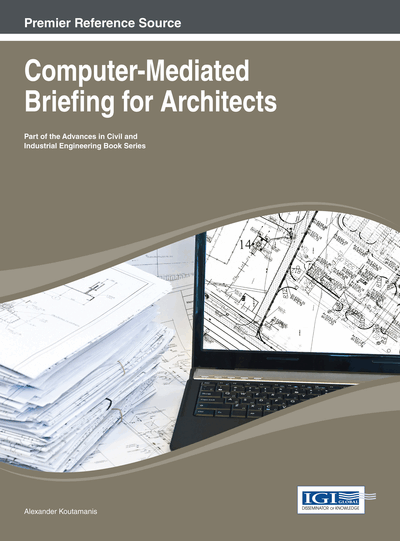# Constraints

DOI: 10.4018/978-1-4666-4647-6.ch009

## Abstract

Constraints and parameterization are powerful design mechanisms but often poorly understood. The chapter starts with a succinct explanation of what they are and what they do in a design context. Then it moves on to the constraint-related facilities available in CAD (AutoCAD) and BIM software (Revit and AutoCAD Architecture), and considers their application with respect to brief constraints on the form, position, and relationships between design entities (spaces and building elements).
Chapter Preview
Top

## Definitions

### Parameterization

Constraints are among the most powerful concepts we can use to make design representations explicit, transparent, and controllable. Recently, constraints have become a popular subject in computer applications like CAD and BIM, in the framework of parametric design. Unfortunately there is some confusion concerning the terms and concepts involved in parameterization. To clarify matters, let us consider a simple geometric example: an equation for describing a straight line is:

y = ax + b

In this equation a and b are parameters. Their values do not change the general type of the graphic entity, as all lines defined by the equation are straight. What these parameters do is change certain line properties: a determines the slope of the line (e.g., if it is 1 the line has a slope of 45°), while b determines the point where the line intersects with the y axis (y-intercept).

Parametric design is founded on this basic structure: rather than having fixed values for the geometry of architectural objects, we can use parametric definitions to change it in a precise manner. For example, moving the line to a new position (translation) can be achieved by just changing the value of b, as presented in Figure 1.

By manipulating parameter values, we can simplify the modification of geometric properties of objects in digital representations (e.g., move an object by changing the value of its distance from a specified point), make multiple copies of the same prototype, and adjust their orientation and position (e.g., create a rectangular array of load-bearing columns and change the distance between columns by manipulating the values of just two parameters, one for the horizontal and one for the vertical spacing). However, the most popular application of parametric design is the transformation of complex geometries to create variations of the same basic form, for instance, the modification of curved surfaces by tweaking parameters so as to change curvature or smoothness. In all cases, the main advantage of parameterization is that it allows control of the design through a few parameter values, which can be quite economical and transparent.

## Complete Chapter List

Search this Book:
Reset